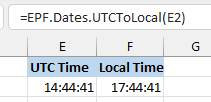# Miscellaneous Formulas

Excel Price Feed currently includes one additional formula which is neither data provider nor instrument specific.

As we add more useful formuals to the software we will document them here.

#### EPF.Dates.UTCToLocal

This formula is used to convert from a UTC (Coordinated Universal Time) date/time to a local date/time.

Most of the date/times used throughout the Add-in are in UTC format and this formula is useful for converting any UTC date/time to the date/time in the timezone of your PC/Excel.

For example, the formula below will convert a UTC time of 14:44 (in cell E2) to the local time on my PC, in this case 17:44 (currently a 3 hour offset):

`=EPF.Dates.UTCToLocal(E2)`This formula, like any regular Excel formula, can take another formula as input.

For example we can use the regular market time fomrula (in UTC) as an input to this formula to convert it to local time:

`=EPF.Dates.UTCToLocal(EPF.Yahoo.RegularMarketTime("AAPL"))`